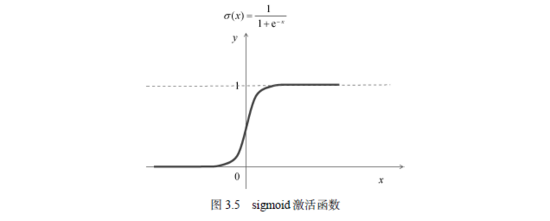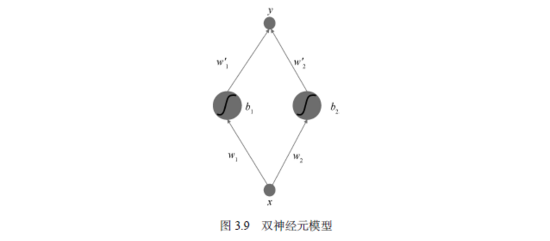﻿ 运用PyTorch动手搭建一个共享单车预测器_python_脚本之家
python# 运用PyTorch动手搭建一个共享单车预测器

3.1 共享单车的烦恼

www.capitalbikeshare.com/ system-data 。3.2 单车预测器1.0

3.2.1 人工神经网络简介3.2.2 人工神经元x表示输入的数据，y表示输出的数据，它们都是实数。从输入单元到隐含层的权重w、隐含层单元偏置b、隐含层到输出层的权重w'都是可以任意取值的实数。3.2.3 两个隐含层神经元3.2.4 训练与运行3.2.5 失败的神经预测器```import numpy as np
import pandas as pd #读取csv文件的库
import torch
import torch.optim as optim
import matplotlib.pyplot as plt
#让输出图形直接在Notebook中显示
%matplotlib inline```

```data_path = 'hour.csv' #读取数据到内存，rides为一个dataframe对象
counts = rides['cnt'][:50] #截取数据
x = np.arange(len(counts)) #获取变量x
y = np.array(counts) #单车数量为y
plt.figure(figsize = (10, 7)) #设定绘图窗口大小
plt.plot(x, y, 'o-') #绘制原始数据
plt.xlabel('X') #更改坐标轴标注
plt.ylabel('Y') #更改坐标轴标注```

```#输入变量，1,2,3,...这样的一维数组
x = Variable(torch.FloatTensor(np.arange(len(counts), dtype = float)))
#输出变量，它是从数据counts中读取的每一时刻的单车数，共50个数据点的一维数组，作为标准答案
y = Variable(torch.FloatTensor(np.array(counts, dtype = float)))

sz = 10 #设置隐含层神经元的数量
#初始化输入层到隐含层的权重矩阵，它的尺寸是(1,10)
weights = Variable(torch.randn(1, sz), requires_grad = True)
#初始化隐含层节点的偏置向量，它是尺寸为10的一维向量
biases = Variable(torch.randn(sz), requires_grad = True)
#初始化从隐含层到输出层的权重矩阵，它的尺寸是(10,1)
weights2 = Variable(torch.randn(sz, 1), requires_grad = True) ```

```learning_rate = 0.0001 #设置学习率
losses = [] #该数组记录每一次迭代的损失函数值，以方便后续绘图
for i in range(1000000):
#从输入层到隐含层的计算
hidden = x.expand(sz, len(x)).t() * weights.expand(len(x), sz) + biases.expand(len(x), sz)
#此时，hidden变量的尺寸是：(50,10)，即50个数据点，10个隐含层神经元

#将sigmoid函数作用在隐含层的每一个神经元上
hidden = torch.sigmoid(hidden)
#隐含层输出到输出层，计算得到最终预测
predictions = hidden.mm(weights2)
#此时，predictions的尺寸为：(50,1)，即50个数据点的预测数值
#通过与数据中的标准答案y做比较，计算均方误差
loss = torch.mean((predictions - y) ** 2)
#此时，loss为一个标量，即一个数
losses.append(loss.data.numpy())

if i % 10000 == 0: #每隔10000个周期打印一下损失函数数值
print('loss:', loss)

#*****************************************
#接下来开始梯度下降算法，将误差反向传播
loss.backward() #对损失函数进行梯度反传

#利用上一步计算中得到的weights，biases等梯度信息更新weights或biases的数值

#清空所有变量的梯度值

```plt.plot(losses)
plt.xlabel('Epoch')
plt.ylabel('Loss')``````x_data = x.data.numpy() #获得x包裹的数据
plt.figure(figsize = (10, 7)) #设定绘图窗口大小
xplot, = plt.plot(x_data, y.data.numpy(), 'o') #绘制原始数据
yplot, = plt.plot(x_data, predictions.data.numpy()) #绘制拟合数据
plt.xlabel('X') #更改坐标轴标注
plt.ylabel('Y') #更改坐标轴标注
plt.legend([xplot, yplot],['Data', 'Prediction under 1000000 epochs']) #绘制图例
plt.show()````x = Variable(torch.FloatTensor(np.arange(len(counts), dtype = float) / len(counts)))````counts_predict = rides['cnt'][50:100] #读取待预测的后面50个数据点
x = Variable(torch.FloatTensor((np.arange(len(counts_predict), dtype = float) + len(counts)) / len(counts)))
#读取后面50个点的y数值，不需要做归一化
y = Variable(torch.FloatTensor(np.array(counts_predict, dtype = float)))

#用x预测y
hidden = x.expand(sz, len(x)).t() * weights.expand(len(x), sz) #从输入层到隐含层的计算
hidden = torch.sigmoid(hidden) #将sigmoid函数作用在隐含层的每一个神经元上
predictions = hidden.mm(weights2) #从隐含层输出到输出层，计算得到最终预测
loss = torch.mean((predictions - y) ** 2) #计算预测数据上的损失函数
print(loss)

#将预测曲线绘制出来
x_data = x.data.numpy() #获得x包裹的数据
plt.figure(figsize = (10, 7)) #设定绘图窗口大小
xplot, = plt.plot(x_data, y.data.numpy(), 'o') #绘制原始数据
yplot, = plt.plot(x_data, predictions.data.numpy()) #绘制拟合数据
plt.xlabel('X') #更改坐标轴标注
plt.ylabel('Y') #更改坐标轴标注
plt.legend([xplot, yplot],['Data', 'Prediction']) #绘制图例
plt.show()```3.2.6 过拟合

3.3 单车预测器2.0

3.3.1 数据的预处理过程

1. 类型变量的独热编码```dummy_fields = ['season', 'weathersit', 'mnth', 'hr', 'weekday'] #所有类型编码变量的名称
for each in dummy_fields:
#取出所有类型变量，并将它们转变为独热编码
dummies = pd.get_dummies(rides[each], prefix=each, drop_first=False)
#将新的独热编码变量与原有的所有变量合并到一起
rides = pd.concat([rides, dummies], axis=1)

#将原来的类型变量从数据表中删除
fields_to_drop = ['instant', 'dteday', 'season', 'weathersit', 'weekday', 'atemp', 'mnth', 'workingday',
'hr'] #要删除的类型变量的名称
data = rides.drop(fields_to_drop, axis=1) #将它们从数据库的变量中删除```** 2. 数值类型变量的处理**temp'是一个位于[-1, 1]区间的数。这样做的好处就是可以将不同取值范围的变量设置为处于平等的地位。

```quant_features = ['cnt', 'temp', 'hum', 'windspeed'] #数值类型变量的名称
scaled_features = {} #将每一个变量的均值和方差都存储到scaled_features变量中
for each in quant_features:
#计算这些变量的均值和方差
mean, std = data[each].mean(), data[each].std()
scaled_features[each] = [mean, std]
#对每一个变量进行归一化
data.loc[:, each] = (data[each] - mean)/std```

** 3. 数据集的划分**```test_data = data[-21*24:] #选出训练集
train_data = data[:-21*24] #选出测试集

#目标列包含的字段
target_fields = ['cnt','casual', 'registered']

#训练集划分成特征变量列和目标特征列
features, targets = train_data.drop(target_fields, axis=1), train_data[target_fields]

#测试集划分成特征变量列和目标特征列
test_features, test_targets = test_data.drop(target_fields, axis=1), test_data[target_fields]

#将数据类型转换为NumPy数组
X = features.values #将数据从pandas dataframe转换为NumPy
Y = targets['cnt'].values
Y = Y.astype(float)

Y = np.reshape(Y, [len(Y),1])
losses = []```

3.3.2 构建神经网络```#定义神经网络架构，features.shape个输入层单元，10个隐含层，1个输出层
input_size = features.shape
hidden_size = 10
output_size = 1
batch_size = 128
neu = torch.nn.Sequential(
torch.nn.Linear(input_size, hidden_size),
torch.nn.Sigmoid(),
torch.nn.Linear(hidden_size, output_size),
)```

`cost = torch.nn.MSELoss()`

`optimizer = torch.optim.SGD(neu.parameters(), lr = 0.01)`

`torch.optim.SGD()`调用了PyTorch自带的随机梯度下降算法（stochastic gradient descent，SGD）作为优化器。在初始化optimizer的时候，我们需要待优化的所有参数（在本例中，传入的参数包括神经网络neu包含的所有权重和偏置，即`neu.parameters()），`以及执行梯度下降算法的学习率lr=0.01。在一切材料都准备好之后，我们便可以实施训练了。```#神经网络训练循环
losses = []
for i in range(1000):
#每128个样本点被划分为一批，在循环的时候一批一批地读取
batch_loss = []
#start和end分别是提取一批数据的起始和终止下标
for start in range(0, len(X), batch_size):
end = start + batch_size if start + batch_size < len(X) else len(X)
xx = Variable(torch.FloatTensor(X[start:end]))
yy = Variable(torch.FloatTensor(Y[start:end]))
predict = neu(xx)
loss = cost(predict, yy)
loss.backward()
optimizer.step()
batch_loss.append(loss.data.numpy())

#每隔100步输出损失值
if i % 100==0:
losses.append(np.mean(batch_loss))
print(i, np.mean(batch_loss))

#打印输出损失值
plt.plot(np.arange(len(losses))*100,losses)
plt.xlabel('epoch')
plt.ylabel('MSE')```3.3.3 测试神经网络

```targets = test_targets['cnt'] #读取测试集的cnt数值
targets = targets.values.reshape([len(targets),1]) #将数据转换成合适的tensor形式
targets = targets.astype(float) #保证数据为实数

#将特征变量和目标变量包裹在Variable型变量中
x = Variable(torch.FloatTensor(test_features.values))
y = Variable(torch.FloatTensor(targets))

#用神经网络进行预测
predict = neu(x)
predict = predict.data.numpy()

fig, ax = plt.subplots(figsize = (10, 7))

mean, std = scaled_features['cnt']
ax.plot(predict * std + mean, label='Prediction')
ax.plot(targets * std + mean, label='Data')
ax.legend()
ax.set_xlabel('Date-time')
ax.set_ylabel('Counts')
dates = pd.to_datetime(rides.loc[test_data.index]['dteday'])
dates = dates.apply(lambda d: d.strftime('%b %d'))
ax.set_xticks(np.arange(len(dates))[12::24])
_ = ax.set_xticklabels(dates[12::24], rotation=45)```3.4 剖析神经网络Neu

```def feature(X, net):
#定义一个函数，用于提取网络的权重信息，所有的网络参数信息全部存储在neu的named_parameters集合中
X = Variable(torch.from_numpy(X).type(torch.FloatTensor), requires_grad = False)
dic = dict(net.named_parameters()) #提取这个集合
weights = dic['0.weight'] #可以按照“层数.名称”来索引集合中的相应参数值
biases = dic['0.bias']
h = torch.sigmoid(X.mm(weights.t()) + biases.expand([len(X), len(biases)])) #隐含层的计算过程
return h #输出层的计算```

```bool1 = rides['dteday'] == '2012-12-22'
bool2 = rides['dteday'] == '2012-12-23'
bool3 = rides['dteday'] == '2012-12-24'

#将3个布尔型数组求与
bools = [any(tup) for tup in zip(bool1,bool2,bool3) ]
#将相应的变量取出来
subset = test_features.loc[rides[bools].index]
subtargets = test_targets.loc[rides[bools].index]
subtargets = subtargets['cnt']
subtargets = subtargets.values.reshape([len(subtargets),1])```

```#将数据输入到神经网络中，读取隐含层神经元的激活数值，存入results中
results = feature(subset.values, neu).data.numpy()
#这些数据对应的预测值（输出层）
predict = neu(Variable(torch.FloatTensor(subset.values))).data.numpy()
#将预测值还原为原始数据的数值范围
mean, std = scaled_features['cnt']
predict = predict * std + mean
subtargets = subtargets * std + mean```

```#将所有的神经元激活水平画在同一张图上
fig, ax = plt.subplots(figsize = (8, 6))
ax.plot(results[:,:],'.:',alpha = 0.1)
ax.plot((predict - min(predict)) / (max(predict) - min(predict)),'bo-',label='Prediction')
ax.plot((subtargets - min(predict)) / (max(predict) - min(predict)),'ro-',label='Real')
ax.plot(results[:, 6],'.:',alpha=1,label='Neuro 7')

ax.set_xlim(right=len(predict))
ax.legend()
plt.ylabel('Normalized Values')

dates = pd.to_datetime(rides.loc[subset.index]['dteday'])
dates = dates.apply(lambda d: d.strftime('%b %d'))
ax.set_xticks(np.arange(len(dates))[12::24])
_ = ax.set_xticklabels(dates[12::24], rotation=45)``````#找到与峰值对应的神经元，将其到输入层的权重输出
dic = dict(neu.named_parameters())
weights = dic['0.weight']
plt.plot(weights.data.numpy()[6, :],'o-')
plt.xlabel('Input Neurons')
plt.ylabel('Weight')```3.5 小结

3.6 Q&A

Q：神经元是不是越多越好？

A：当然不是越多越好。神经网络模型的预测能力不只和神经元的个数有关，还与神经网络的结构和输入数据有关。

Q：在预测共享单车使用量的实验中，为什么要做梯度清空？

A：如果不清空梯度，backward()函数是会累加梯度的。我们在进行一次训练后，就立即进行梯度反传，所以不需要系统累加梯度。如果不清空梯度，有可能导致模型无法收敛。

Q：对于神经网络来说，非收敛函数也可以逼近吗？

A：在一定的闭区间里是可以的。因为在闭区间里，一个函数不可能无穷发散，总会有一个界限，那么就可以使用神经网络模型进行逼近。对于一个无穷的区间来说，神经网络模型就不行了，因为神经网络模型中用于拟合的神经元数量是有限的。

Q：在预测共享单车的例子中，模型对圣诞节期间的单车使用量预测得不够准确。那么是不是可以通过增加训练数据的方法提高神经网络预测的准确性？

A：是可行的。如果使用更多的包含圣诞节期间单车使用情况的训练数据训练模型，那么模型对圣诞节期间的单车使用情况的预测会更加准确。

Q：既然预测共享单车使用量的模型可以被解析和剖析，那么是不是每个神经网络都可以这样剖析？

A：这个不一定。因为预测共享单车使用量的模型结构比较简单，隐藏层神经元只有10个。当网络模型中神经元的个数较多或者有多层神经元的时候，神经网络模型的某个“决策”会难以归因到单个神经元里。这时就难以用“剖析”的方式来分析神经网络模型了。

Q：在训练神经网络模型的时候，讲到了“训练集/测试集=k”，那么比例k是多少才合理，k对预测的收敛速度和误差有影响吗？

A：在数据量比较少的情况下，我们一般按照10∶1的比例来选择测试集；而在数据量比较大的情况下，比如，数据有十万条以上，就不一定必须按照比例来划分训练集和测试集了。